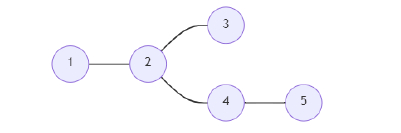# C++ Program to find out the super vertices in a graph

Suppose, we are given a graph that has n vertices. The vertices are numbered 1 to n, and they are connected by the edges given in the array 'edges'. Each vertex has an 'x' value within a number from 1 to n that is given in the array 'values'. Now, we have to find out the super vertices from the graph. A vertex i is called a 'super vertex' whenever the shortest path from vertex 1 to i doesn't have a vertex with the same 'x' value as the i-th vertex. We print out all the vertices satisfying this criterion.So, if the input is like n = 5, values = {1, 2, 2, 1, 3}, edges = {{1, 2}, {2, 3}, {2, 3}, {2, 4}, {4, 5}}, then the output will be 1 3 4 5.

Every vertex except vertex 2 satisfies the criterion. So, vertex 2 is excluded.

## Steps

To solve this, we will follow these steps −

Define arrays vertexVal, frq, and chk of size: 100005.
Define an array vcti of size 200005.
Define a function dfs(), this will take j, k,
if frq[vertexVal[j]] is same as 0, then:
chk[j] := 1
(increase frq[vertexVal[j]] by 1)
for each value a in vcti[j], do:
if a is not equal to k, then:
dfs(a, j)
(decrease frq[vertexVal[j]] by 1)
for initialize i := 0, when i < n, update (increase i by 1), do:
vertexVal[i] := values[i]
for initialize i := 0, when i < n, update (increase i by 1), do:
a := first value of edges[i]
b := second value of edges[i]
insert b at the end of vcti[a]
insert a at the end of vcti[b]
dfs(1, 0)
for initialize i := 1, when i <= n, update (increase i by 1), do:
if chk[i] is non-zero, then:
print(i)

## Example

Let us see the following implementation to get better understanding −

#include <bits/stdc++.h>
using namespace std;

int n;
int vertexVal, frq, chk;
vector<int> vcti;

void dfs(int j, int k){
if (frq[vertexVal[j]] == 0)
chk[j] = 1;
frq[vertexVal[j]]++;
for (auto a : vcti[j]) {
if (a != k)
dfs(a, j);
}
frq[vertexVal[j]]--;
}
void solve(int values[], vector<pair<int, int>> edges){
for (int i = 0; i < n; i++)
vertexVal[i] = values[i];
for (int i = 0; i < n; i++){
int a, b;
a = edges[i].first;
b = edges[i].second;
vcti[a].push_back(b);
vcti[b].push_back(a);
}
dfs(1, 0);
for (int i = 1;i <= n; i++){
if (chk[i]) cout<< i <<endl;
}
}
int main() {
n = 5;
int values[] = {1, 2, 2, 1, 3}; vector<pair<int, int>> edges = {{1, 2}, {2, 3}, {2, 3}, {2, 4}, {4, 5}};
solve(values, edges);
return 0;
}

## Input

5, {1, 2, 2, 1, 3}, {{1, 2}, {2, 3}, {2, 3}, {2, 4}, {4, 5}}

## Output

1
3
4
5